Function Repository Resource:

# VertexArc

Graphics primitive for the arc of a polygon vertex

Contributed by: Ed Pegg Jr and Eric Weisstein
 ResourceFunction["VertexArc"][{q1,p,q2},rad] constructs a circular arc centered at p with radius rad from vertex points p,q1 and q2.

## Examples

### Basic Examples (2)

Form a vertex arc:

 In:=Out=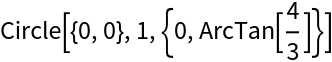Show the arc and vertex:

 In:=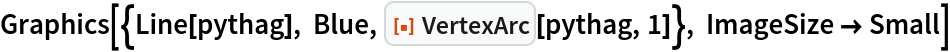Out=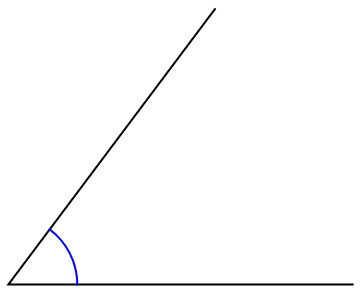### Scope (3)

Four non-congruent triangles with three shared angles and various shared sides:

 In:=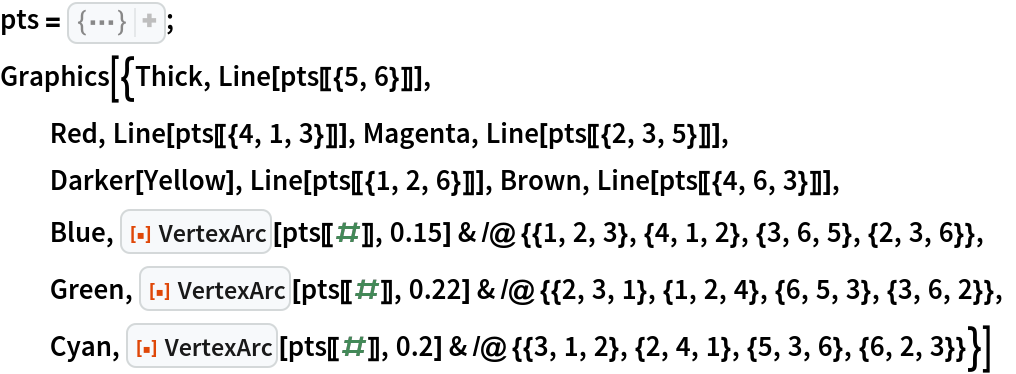Out=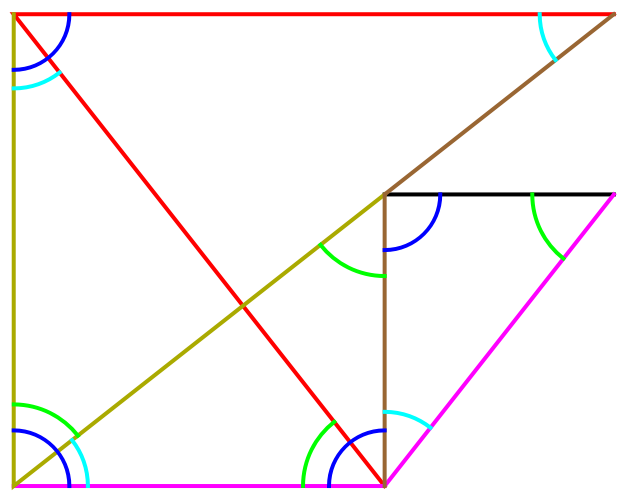A rectangle dissected into seven similar triangles:

 In:=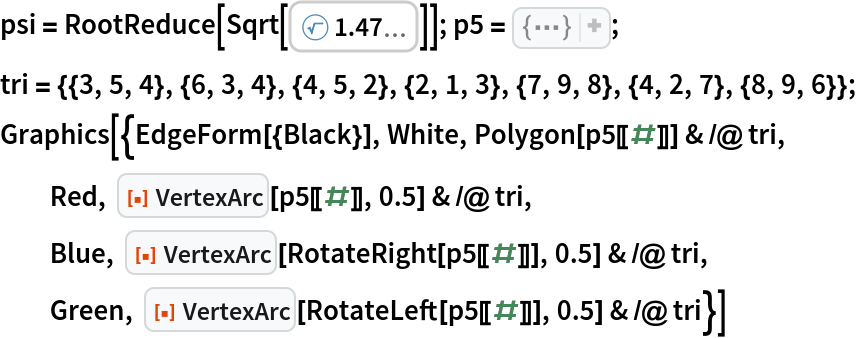Out=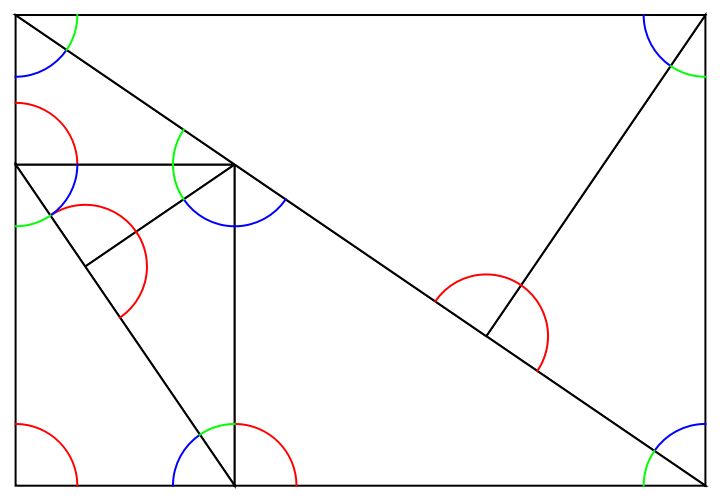In:=Out=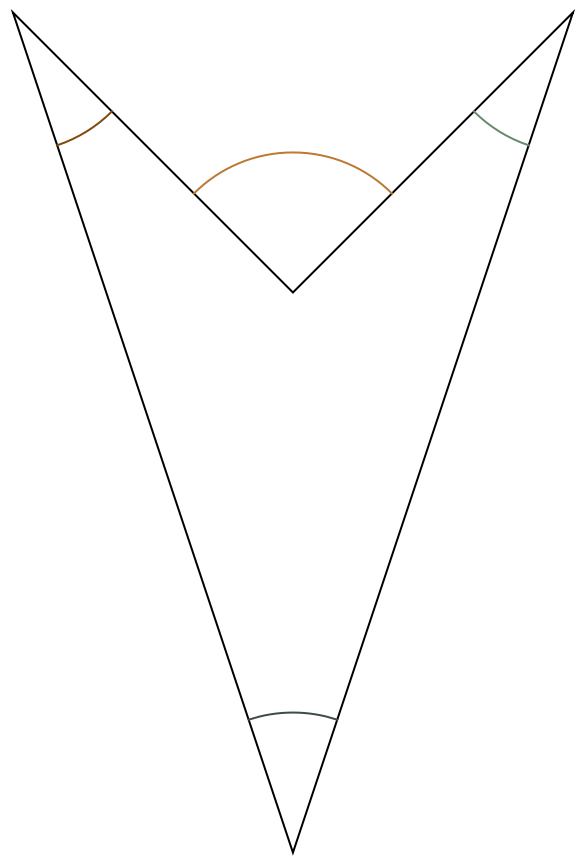### Possible Issues (1)

For a 180°, the resulting arc is arbitrarily oriented:

 In:=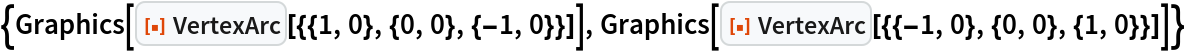Out=### Neat Examples (1)

In the psi-quad substitution tiling,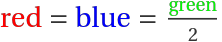:

 In:=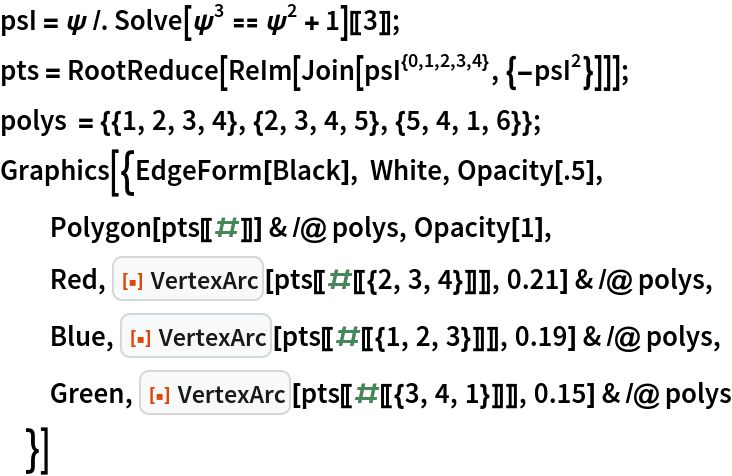Out=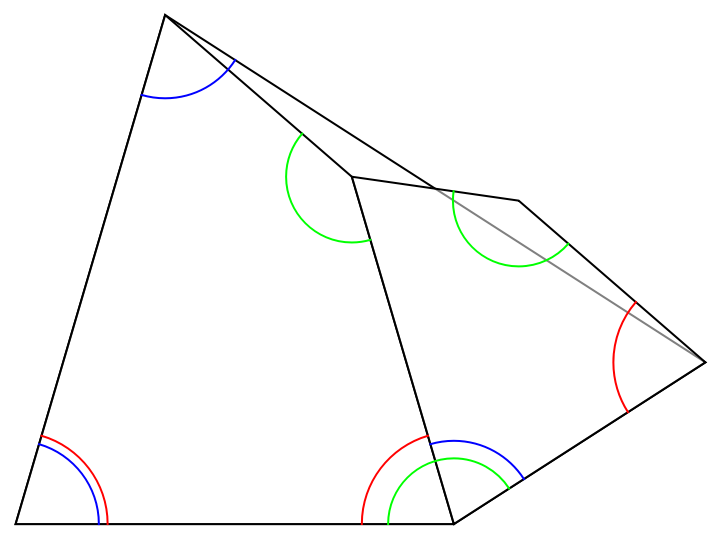## Version History

• 1.0.0 – 03 May 2022